Search company, investor...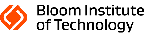# Bloom Institute of Technology

bloomtech.com

2017

## Stage

Unattributed VC | Alive

## Total Raised

134.19M ## About Bloom Institute of Technology Bloom Institute of Technology operates as an online coding platform. It offers vocational training with courses on software engineering and data science. The company enables students to gain hands-on training and skills to find placement opportunities. The company was formerly known as Lambda School. It was founded in 2017 and is based in San Francisco, California. ## Headquarters Location 548 Market Street PMB 69148 San Francisco, California, 94104, United States 800-883-1943Looking for a leg up on competitive, customer and technology insights? CB Insights puts confidence and clarity into your most strategic decisions. See how. Join a demo.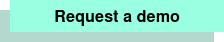Trusted by the world's smartest companies to: • Predict emerging trends • See competitors' playbooks • Stalk the smart money • Identify tomorrow's challengers • Spot growing industries • Kill analyst data work Let's see how we can help you!You're one click away from the most comprehensive, unmatched analyst expertise in tech, in-depth private company data and a platform that brings it all together. Click Now. Join a live demo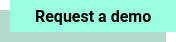## Research containing Bloom Institute of Technology Get data-driven expert analysis from the CB Insights Intelligence Unit. CB Insights Intelligence Analysts have mentioned Bloom Institute of Technology in 2 CB Insights research briefs, most recently on Feb 25, 2021.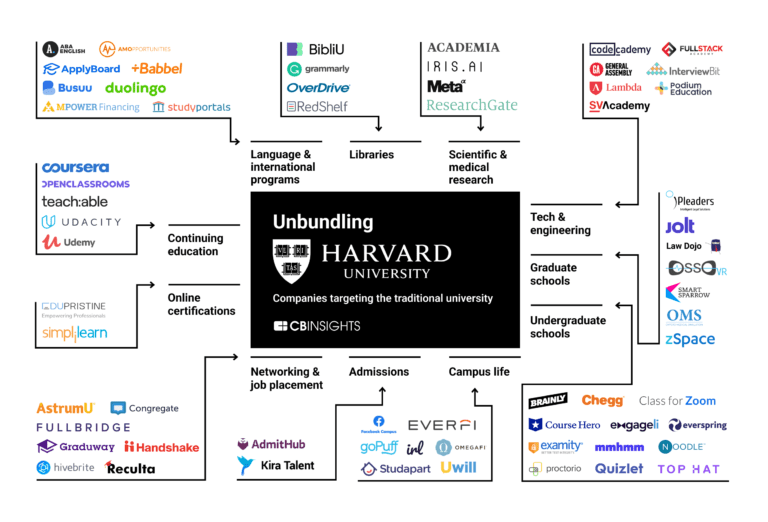## Expert Collections containing Bloom Institute of Technology Expert Collections are analyst-curated lists that highlight the companies you need to know in the most important technology spaces. Bloom Institute of Technology is included in 1 Expert Collection, including Education Technology (Edtech). E # Education Technology (Edtech) 2,797 items These companies offer tech-enabled solutions that facilitate education and learning for people of all ages, from pre-K to adult and professional education. ## Latest Bloom Institute of Technology News A framework for Li-ion battery prognosis based on hybrid Bayesian physics-informed neural networks Aug 24, 2023 Abstract Li-ion batteries are the main power source used in electric propulsion applications (e.g., electric cars, unmanned aerial vehicles, and advanced air mobility aircraft). Analytics-based monitoring and forecasting for metrics such as state of charge and state of health based on battery-specific usage data are critical to ensure high reliability levels. However, the complex electrochemistry that governs battery operation leads to computationally expensive physics-based models; which become unsuitable for prognosis and health management applications. We propose a hybrid physics-informed machine learning approach that simulates dynamical responses by directly implementing numerical integration of principle-based governing equations through recurrent neural networks. While reduced-order models describe part of the voltage discharge under constant or variable loading conditions, model-form uncertainty is captured through multi-layer perceptrons and battery-to-battery aleatory uncertainty is modeled through variational multi-layer perceptrons. In addition, we use a Bayesian approach to merge fleet-wide data in the form of priors with battery-specific discharge cycles, where the battery capacity is fully available or only partially available. We illustrate the effectiveness of our proposed framework using the NASA Prognostics Data Repository Battery dataset, which contains experimental discharge data on Li-ion batteries obtained in a controlled environment. Introduction Electric and hybrid-propulsion systems are key enablers of advanced air mobility transformation, in which small and large aircraft will rely on Li-ion batteries to source part of all power needs. As a critical component of the powertrain, safe operation of these batteries will require robust prognosis and health management methods 1 , 2 . Current literature shows an array of methods for battery monitoring with models based on first principles 3 , 4 , machine learning 5 , 6 , 7 , and a combination of both 8 , 9 , 10 . However, existing modeling approaches often find roadblocks including: (a) governing equations are complex; and when available, high-fidelity simulations are computationally expensive to be executed onboard; (b) purely data-driven models do not necessarily obey the governing physics, nor do they generalize well to scenarios on which they have not been trained; and (c) collecting enough high-quality data to adequately train data-driven models for a complex system is often challenging—in fact, data available for adjusting reduced-order models or building machine learning models can be poor (plagued with noise, missing data, unbalanced observations of inputs and outputs, etc.). These challenges are commonly shared across many prognosis applications; creating a need for a robust modeling approach that is computationally efficient, while being grounded on first principles, and can account for unstructured datasets. With this background, physics-informed neural networks 11 , 12 , 13 have the potential to revolutionize prognosis and health management. This class of machine learning methods can potentially mitigate the lack of data as well as other problems such as poor interpretability of purely data-driven models while offering accuracy that is comparable to high-fidelity simulations at a fraction of the computational cost. In fact, recent developments in neural operators 14 , 15 indicate that for problems in which the partial differential equations are known, the trained neural networks can be reused to make predictions even outside the boundary/initial conditions used in training. However, many complex systems cannot be described purely by partial differential equations, but rather by a set of governing equations and empirical laws that might not be fully characterized at the same time that available data are scarce. This explains the growing interest in hybrid physics-informed machine learning 16 , 17 as a promising modeling framework for complex applications such as electric and hybrid propulsion systems. The hybrid framework we propose uses a different paradigm when compared to physics-driven loss functions as in 11 . It leverages existing equations of a system to build a model, and introduces small data-driven kernels strategically within the model. The data-driven portions of the model compensate for missing physics, model-form uncertainty, and ignorance of model parameters. Figure 1 Hybrid Bayesian physics-informed neural network framework and its application to prognosis of Li-ion batteries. (a) Our framework directly implements numerical integration of governing equations through a hybrid graph model that merges principle-based nodes, data-driven nodes, and variational Bayesian nodes. As a result, the model can accommodate information coming from design, testbed experiments, historical and usage data in applications ranging from diagnosis and prognosis modeling, design under uncertainty, robust control, etc. (b) In the Li-ion battery prognosis application, the state-of-charge dynamics is modeled through a recurrent neural network such that physics-derived reduced-order models are built in as nodes; and data-driven as well as variational Bayesian nodes quantify the different forms of uncertainty in prediction. Our model can be used for discharge prediction as well as battery aging forecast far ahead in the life of the battery. In terms of Li-ion battery prognosis, it is difficult to build models that can predict battery end of discharge while accurately accounting for the effect of battery aging. Challenges include effect of random-discharge cycles, effect of cumulative energy drawn from the battery, inter-battery variability, temperature effects during aging, and availability/completeness of recorded data. In order for prognosis models to be useful, they must furnish uncertainty estimates used in optimization of batteries operation as well as guiding model updates. In this paper, we propose a hybrid Bayesian physics-informed neural network approach, depicted in Fig. 1 a, to address the following key challenges: Computational footprint: models used for prognosis have to be computationally efficient, both in terms of memory requirement and speed, as they are often used in embedded applications or to run thousands of “what-if” scenarios. Our proposed hybrid model implements the numerical integration of governing time-dependent dynamics using recurrent neural networks 18 . Partially characterized first-principle models: in many real-world applications, degradation or failure mechanisms are only partially modeled, due to computational complexity or lack of complete knowledge. In our approach, model-form uncertainty introduced by either unknown physics or by reduced-order models is compensated with data-driven nodes placed in the design of the recurrent neural networks 19 , 20 . Unstructured datasets: ideally, prognosis models should be able to leverage very diverse sources of information—from legacy systems, to design, to early operation, etc. Our framework can handle heterogeneous sources of data such as design and laboratory experiments (e.g., reduced-order model and constants used therein); while also compensating for reduced number of data points with prior information coming from historical data (e.g., legacy fleet data). The hybrid graph model described in Fig. 1 a is at the foundation of the work presented here. It is composed of a series of nodes, each one corresponding to a single or multiple sets of equations. Such equations can be derived from first principles, empirical or phenomenological models, or data-driven models like deep neural networks and variational Bayesian models. Input–output relationships among the nodes are represented by edges connecting those nodes, thus blending physics-derived and data-driven blocks. Physics-derived model parameters and neural network parameters are then trained in a single stage using off-the-shelf deep learning libraries. The incorporation of different data sources is possible, in part due to the hybrid nature of our model, which mixes principle-based and machine learning kernels. For example, the principle-based portion of the model is friendly to engineering and design information; while the machine learning portion can accommodate observed data. In addition, our Bayesian approach to data fusion makes it possible to use data that becomes available in different points during the battery life cycle. In recent years, hybrid and physics-informed data-driven modeling strategies for prognostics have been proposed. Some 21 , 22 use a mixture of physics-based models or parameters derived experimentally to feed neural networks with the intent to predict the remaining useful life of complex systems, and potentially outperform pure data-driven models. Others 23 proposed a deep learning architecture where the last layer is tailored to solve differential equations to compute the point-solution at two time steps simultaneously, to estimate parameters of power electronic converters. An overview of methods that broadly apply to prognostics, reliability analysis as well as system safety can be found in 24 . Our prognostics framework differs from other, existing frameworks as it blends physics-derived equations and data-driven kernels in a sole hybrid model, and can train both data-driven and physical parameters together using back-propagation. Other frameworks combine physics and machine learning sequentially, where each model is calibrated/trained independently and serves as input to the other. Our framework can also accommodate physics-driven error metrics in the loss function like in 11 . In addition, model parameters can either be trained for each physical sample, e.g., train a different parameter for each battery in the training set separately, or shared across multiple samples of the same system type, e.g., train parameters to represent a fleet of similar batteries. A single model can be composed of a mix of both, parameters that are shared across different specimens, and parameters that needs to be tailored for each specimen. Figure 1 b details the application of our proposed framework to the prognosis of Li-ion batteries. The battery state of charge is approximated using discretized ordinary differential equations based on the Nerst and Butler–Volmer models 25 , 26 . These are at the foundation of the principle-based nodes (blue blocks), which captures the major trend of the battery state-of-charge, but discrepancies between the predictions of those models and field data prevent them from being used alone for battery risk management. Therefore, we pair the principle-based nodes in the graph with (a) purely data-driven nodes to adjust for model-form uncertainty coming from model simplifications; and (b) variational Bayesian nodes 27 , 28 , 29 to account for data uncertainty coming from battery-to-battery variability and observation uncertainty. In practical terms, our hybrid model offers the following key benefits to battery operators: (1) it does not rely solely on constant discharge curves, which are the standard to estimate the battery residual capacity; and therefore, model updating can be performed without decommission of the battery; (2) it can handle battery-to-battery variation; which can happen due to factors such as inherent variability of manufacturing, initial internal damage, etc; and (3) it models battery degradation incorporating fleet-wide data; and with the aid of the Bayesian formulation, it can handle model updates with full and partial discharge cycles as well as missing history of battery usage. The approach is demonstrated using the experimental data publicly available through the NASA Prognostics Center of Excellence Data Repository 30 , 31 . Results We present four key results: (1) estimation of model-form inadequacy in the state-of-charge model; (2) modeling of battery aging with incorporation of battery-to-battery variation; (3) model update using full and partial battery discharge cycles; and (4) ability to derive battery-specific models without requiring battery usage history. Handling model-form uncertainty with hybrid physics-informed neural network The first result of the proposed hybrid physics-informed neural network is that the numerical integration of ordinary differential equations governing the state of charge is directly implemented as a recurrent neural network. This class of network models the one-step-ahead prediction of the response of interest and associated model states, given set of input values: \begin{aligned}{}[ {\textbf{y}}_t \quad {\textbf{h}}_t ]^{\top } = f({\textbf{u}}_t, {\textbf{y}}_{t-1}, {\textbf{h}}_{t-1}), \end{aligned} (1) where the subscript t represents the time discretization; $${\textbf{y}} \in {\mathrm{I\!R}}^{n_{y}}$$ are the observable responses; $${\textbf{h}} \in {\mathrm{I\!R}}^{n_{h}}$$ are the internal states; $${\textbf{u}} \in {\mathrm{I\!R}}^{n_{u}}$$ are input variables; and f(.) defines the transition between time steps. Figure 2 Implementing a hybrid physics-informed neural network for Li-ion prognosis. (a) Recurrent neural network design implements numerical integration of governing equations in the state-space representation. The recurrent unit is composed by surrogate models describing the main phenomena driving the battery electrochemistry, a data-driven node that captures the non-ideal internal voltage, and a variational Bayesian node that models aging through degradation of battery parameters. (b) The hybrid model takes a current time series as input and returns battery voltage. This allows one to use the model for tracking of present discharge cycles as well as forecast of future missions. (c) Comparison between experimental data and predictions out of our hybrid physics-informed neural network. Predictions are obtained for batteries not used in the training set. As detailed in Fig. 2 a, we propose designing f(.) in Eq. ( 1 ) so that (i) we implement the appropriate integration scheme 32 (e.g., Euler, Runge-Kutta, etc.) for the set of governing equations; (ii) we use principle-based models with the intent to capture trends in the data; and (iii) we add data-driven and variational kernels to quantify model uncertainty. For the Li-ion battery model, the physics-based nodes implement the simplified electrochemistry given by the Butler–Volmer–Nernst models. The data-driven nodes estimate the non-ideal internal voltage $$V_{ni, i},\,\,i=\{p. n\}$$, while the variational Bayesian nodes model the total resistance $$R_0$$ (Ohms) and the maximum charge $$q^{max}$$ (Coulombs), which values are driven by the aging of the battery and may change from one specimen to another. Therefore, the data-driven and variational Bayesian nodes characterize epistemic and aleatory uncertainties in the principle-based models. The computational cost associated with our model is remarkably low. The principle-based models come from fast-to-compute reduced order models and the data-driven models are shallow Multi-Layer Perceptrons (MLPs) and variational Multi-Layer Perceptrons (vMLPs). The first-order ordinary differential equation that governs the time-dependent response is integrated using a state-space representation and is ideal for onboard computing or massive uncertainty quantification offline. Details about the implementation of the nodes are given in the Methods section and the Supplementary Material illustrate the costs associated with training and prediction. Figure 2 b illustrates the use of our hybrid models. A time series with the electric current drawn from the battery is used as input. At each time step, the hybrid physics-informed neural network updates the internal states as well as estimates the values for the voltage drop between the battery terminals. The set of hyperparameters of the hybrid model for both data-driven and variational Bayesian nodes are estimated using a set of discharge cycles. Full discharge cycles performed with fresh batteries are used in the parameter optimization of the data-driven model used to describe the non-ideal internal voltage (aging models utilize, instead, both full and partial discharge cycles). In order to ensure diversity, the training data comes from a set of batteries exposed to different duty cycles. Finally, the trained model can then be used to predict battery discharge for batteries and missions not present in the training set. Figure 2 c shows how hybrid model predictions compare against a sample of random discharge cycles. These results illustrate the model’s ability to track voltage throughout the cycle. This allows one to determine whether the battery will be able to finish a given mission; or to optimize the deployment of a set of batteries across multiple missions. Accounting for battery aging with variational Bayesian models The second result of our framework is that we are able to build an aging model for a battery being monitored (“test battery”) by leveraging existing knowledge from a fleet of similar batteries. To achieve this goal, we resort to vMLPs. The model is then updated with new data coming from the test battery during operation using Bayesian update. Figure 3 Account for battery aging using variational models. (a) Correlation between $$q^{max}$$ and C against cumulative energy drawn from the battery. (b) Individual aging models for the fleet C(E), which serve as fleet prior and are used to define the aging models $$q^{max}(E)$$, $$R_0(E)$$. (c) Ensemble distribution of $$q^{max}$$ from the fleet prior (orange lines), and posterior distribution (green line and shadowed area) computed by updating the fleet prior with estimates of $$q^{max}$$ visible in black circles. (d) The model “a-posteriori” can predict the future discharge profile at different aging stages of the battery. The prediction from the posterior model (green) is compared against predictions carried out without the fleet prior model. First, we observed very strong correlation between capacity fading in aging batteries and changes in model parameters $$q^{max}$$ and $$R_0$$ 19 , 26 , as shown in Fig. 3 a and Fig. S4b of the Supplementary Material. We assumed that historical capacity-fading data from a fleet of older batteries can be collected and used to build aging models for each battery in that fleet. The models aim at predicting the expected value and the confidence intervals as a function of the cumulative energy drawn E, C(E). Each model is composed of two vMLPs to capture epistemic and aleatory uncertainty as described in the Methods section. Figure 3 b shows the output of the individual variational models on top of collected capacity data. Thanks to the strong correlation observed between C and parameters $$q^{max}$$, $$R_0$$, we built an aging model describing $$q^{max}(E)$$ and $$R_o(E)$$, as explained in further details in the Methods section. The variational models of all other batteries in the fleet work as prior for the test battery, and they are combined in an ensemble model as follows: \begin{aligned} q^{max}(E) = \sum _k \omega _k^{q} \, q^{max}_k(E) \qquad {\text {and}} \qquad R_0(E) = \sum _k \omega _k^{R} \, R_{0,k}(E), \end{aligned} (2) where $$\omega _k^q$$ and $$\omega _R^q$$ are the weights of k-th model, defined later in the Methods section; and $$q^{max}_k(E)$$ and $$R_{0,k}(E)$$ are the k-th vMLPs associated with batteries used to build fleet priors, as illustrated in Fig. 3 b. Therefore, $$q^{max}(E)$$ and $$R_0(E)$$ represent the estimates “a priori” as an ensemble model derived from the fleet. The orange lines in Fig. 3 c show an example of prior for $$q^{max}$$. Every time new data is collected from the operation of the test battery, either through a reference discharge cycle test or by indirectly estimating them, we update the ensemble-model prior given the predictions from the fleet, and update the weights and biases of the test battery vMLP models using Bayes’ rule. This leads to a new a-posteriori model, as depicted in Fig. 3 c, green line and shaded area. This approach allows us to leverage the existing trends of capacity fading of older batteries to infer future values of $$q^{max}$$, $$R_0$$ for the test battery. Forecast of $$q^{max}$$, $$R_0$$ at future values of E as the one in Fig. 3 c enables the prediction of the voltage discharge behavior of the test battery at different aging stages, as shown in Fig. 3 d. Dealing with complete and censored data through specialized loss functions and variational models Figure 4 Using full and partial discharge cycles to perform model updates. (a) In partial discharge cycles the only available information is the used capacity; which imposes uncertainty in the estimation of available Li-ions, $$x_{p,n}$$. (b) Fleet information can be used to elucidate prior distributions; while both full and partial discharge cycles can be used for continuous model update. The top panel illustrates the update with one full discharge at $$E = 0 \, {\text{kWh}}$$ and partial discharge cycles between $$E=0 \, {\text{kWh}}$$ and $$E = 1 \, {\text{kWh}}$$. The bottom panel shows the update with full discharges at $$E = 0 \, {\text{kWh}}$$ and $$E = 2 \, {\text{kWh}}$$ and partial discharge cycles between $$E=0 \, {\text{kWh}}$$ and $$E = 2 \, {\text{kWh}}$$. (c) Uncertainty in model parameters can be propagated to state-of-charge throughout a mission (voltage history). Integration of voltage and current is used to estimate power and energy used in each cycle. The estimated energy distribution can be compared against actual used energy. (d) As long as the distribution of the error in the energy estimation is stationary, the hybrid model can be used for the specific battery. Metrics such as KL-divergence can be used to indicate when new full discharge cycles are needed for model updating. The third result of the proposed hybrid physics-informed neural network is the ability to use both complete information (constant discharge) as well as censored data (random discharge) to perform battery-specific model update, as illustrated in Fig. 4 a. Full discharge data are obviously more effective in reducing model-parameter uncertainties than partial discharge data. However, the fact that our framework can incorporate partial discharge implies that the models are updated while the batteries are still in use. From our experiments, we observed that model behavior is consistent with the aging effects observed in the fleet of similar batteries used for training. Thus, if the aging of a test battery follows (in a statistical distribution sense) the aging already seen in the fleet describing the training set, then partial discharge cycles are sufficient to guarantee a proper model updating and refine model predictions of future discharge cycles. However, full discharge data are likely to be necessary for test batteries behaving very differently from the one seen in the training set. The number of full discharge cycles necessary strongly depends on the behavior of the battery during operation. The update of the capacity model for a sample battery is illustrated in Fig. 4 b. The available data at 1 KWh is composed of one constant discharge at 0 KWh and all random discharge cycles run up to 1 KWh. The posterior model can be used to forecast capacity as a function of cumulative energy – Updated Model in Fig. 4 b. Further results on the reduction of uncertainty obtained by updating models with only partial discharge data is provided in the Supplementary Material. In order to evaluate whether a model produces predictions accurate enough for further use, we propose estimating the distribution of the error between the predicted and consumed energy and evaluate the stationarity of such error using a statistical measure. To do so, we predict the voltage discharge curve and corresponding uncertainty coming from the model parameters. Voltage and applied (load) current profile can be integrated to estimate the energy distribution necessary for a specific mission. This energy distribution can then be compared against the actual energy consumed by the battery, as in Fig. 4 c. The models can be used for prognosis for as long as the distribution of this energy error remains stationary. Metrics such as the KL-divergence between the present energy error distribution and the energy error distribution immediately after the model is updated with full discharge data can be used to indicate the need for new full discharge data, as illustrated in Fig. 4 d. The top panel shows the median and the 95% confidence interval of the energy error distribution over the battery usage. While there is expected variation as the battery accumulates cycles, it is not until cumulative energy reaches 2 kWh that the energy error distribution starts to diverge substantially. This is easily captured by KL-divergence, which becomes an index for the “health of the model,” as shown in the bottom panel of Fig. 4 d. The threshold level for the KL-divergence that will indicate the need for new full discharge data is application dependent and we recommend to use a fleet of batteries to determine it. In our study, we found this value to be around 1.0 (see discussion in the Supplementary Material). Quantifying uncertainty in aging due to unknown battery usage through Bayesian update The fourth result of our proposed framework is the ability to perform battery-specific model update without requiring complete knowledge of past battery usage. This scenario is likely to occur when monitoring legacy fleets of battery-powered vehicles and devices. Operators, insurers, and service providers might have to serve batteries with unknown previous operations, route/usage structure and patterns, driver/user behavior, etc. Nevertheless, to control operations and maintenance costs, it is important to quantify battery degradation and estimate capacity fade over future usage. The hybrid physics-informed neural network models for such batteries can be obtained by (1) monitoring present and future decay in capacity against the observed increment in cumulative energy; and (2) performing Bayesian update of fleet-wide model taken as prior information in the light of newly recorded data. Figure 5 Modeling battery aging without known usage history. (a) Although capacity data might be missing for considerable amount of operation, once it is consistently tracked, it can be used for updating aging models. (b) Fleet-wide information of capacity versus cumulative energy is used to estimate cumulative energy distributions for observed capacity levels. (c) Bayesian update is performed on the difference between observed cumulative energy. (d) With enough observed capacity levels, aging models move from fleet to battery-specific distribution. The surface overpotential is described by the Butler–Volmer equation 26 such that \begin{aligned} V = V_{U,p} - V_{U,n} - V_0 - V_{\eta ,p} - V_{\eta , n}, \quad V_{\eta ,i} = \frac{RT}{F\alpha }\,{\textrm{arcsinh}}\left( \frac{J_i}{2J_{i0}}\right) , \quad V_0 = R_0 \,\, i_{app} \end{aligned} (6) where $$\alpha$$ is a symmetry factor, $$J_i$$ is the current density and $$J_{i0}$$ is the exchange current density, while $$R_0$$ is the Ohmic lumped resistance used to represent aging. Non-ideal internal voltage The assumption of unity activity coefficients is not applicable to real batteries; as a result, in this paper, the non-ideal internal voltage is modeled through a multi-layer perceptron (MLP): \begin{aligned} V_{ni, n} = {\textrm{MLP}}_n(x_n; \, \textbf{w}_n, \textbf{b}_n) \quad {\text {and}} \quad V_{ni, p} = {\textrm{MLP}}_p(x_p; \, \textbf{w}_p, \textbf{b}_p), \end{aligned} (7) where $$\textbf{w}_n$$, $$\textbf{b}_n$$, $$\textbf{w}_p$$, and $$\textbf{b}_p$$ are the MLP parameters; and subscripts p and n refer to positive and negative electrode, respectively. The set of equations ( 3 )-( 7 ) define the nodes implemented in the hybrid physics-informed neural network for battery prognostics. Accounting for battery aging with variational models Battery aging is modeled through capacity decay over the useful life, and we use parameters $$q^{max}$$ and $$R_0$$ as proxies for the aging effect on the capacity. The input variable to the aging models is the cumulative energy E drawn from the battery. Both parameters $$q^{max}$$, $$R_0$$ are strongly correlated to C; and thus, we modeled C(E) through: \begin{aligned} {}&C(E) \sim \mathcal {N}(\mu _C(E), \sigma ^2_C(E))\,, \quad q^{max}(E) = \alpha \,C(E), \quad R_0(E) = \frac{q^{max}(E)}{\gamma (E)},\\&\mu _C(E) = \textrm{vMLP}_{\mu _C}(E; \, \textbf{w}_{\mu _C}, \textbf{b}_{\mu _C}) \quad \text {and} \quad \sigma ^2_C(E) = \textrm{vMLP}_{\sigma ^2_C}(E; \, \textbf{w}_{\sigma ^2_C}, \textbf{b}_{\sigma ^2_C}) \end{aligned} (8) where $$\alpha$$ is a scaling factor, determined with the data shown in Fig. 3 a; and $$\gamma (E)$$ is a linear model, reported in the Supplementary Material. The variational models for C(E), and as a consequence for the aging parameters $$q^{max}$$ and $$R_0$$, are composed of one vMLP to predict the mean value of the quantity of interest (epistemic uncertainty), and another one to estimate the confidence interval of the prediction (aleatory uncertainty). Each weight and bias of the vMLPs are described by a normal distribution. This way, for the i-th layer of the $$\mu _C(E)$$ vMLP: \begin{aligned} \textbf{w}_{\mu _C,i} \sim \mathcal {N} \left( \varvec{\mu }_{\textbf{w}_{\mu _C,i}}, \; \varvec{\sigma }^2_{\textbf{w}_{\mu _C,i}} \right) , \quad \textbf{b}_{\mu _C,i} \sim \mathcal {N} \left( \varvec{\mu }_{\textbf{b}_{\mu _C,i}}, \; \varvec{\sigma }^2_{\textbf{b}_{\mu _C,i}} \right) , \end{aligned} (9) and similarly, for the i-th layer of the $$\sigma ^2_C(E)$$ vMLP: \begin{aligned} \textbf{w}_{\sigma _C,i} \sim \mathcal {N} \left( \varvec{\mu }_{\textbf{w}_{\sigma ^2_C,i}}, \; \varvec{\sigma }^2_{\textbf{w}_{\sigma ^2_C,i}} \right) , \quad \textbf{b}_{\sigma _C,i} \sim \mathcal {N} \left( \varvec{\mu }_{\textbf{b}_{\sigma ^2_C,i}}, \; \varvec{\sigma }^2_{\textbf{b}_{\sigma ^2_C,i}} \right) , \end{aligned} (10) The means and standard deviations of these distributions are optimized during training. The loss function is defined in Eq. ( 12 ). Our observation is that aging is best modeled through battery specific $$q^{max}$$ and $$R_0$$. When building a model for a newly deployed battery, such that constant reference discharge data is not enough to build accurate vMLP models; we suggest using an ensemble of fleet-wide models to build priors for the new battery. The ensemble prior and corresponding weights follow: \begin{aligned} C_{WA}(E) = \sum _i \omega _i C_i(E)\; ; \quad \omega _i = \frac{ \Sigma ^{-1} \varvec{1} }{ \varvec{1}^{\top } \Sigma \varvec{1} } \end{aligned} (11) where: $$\Sigma _{i,j} = \varvec{\varepsilon }_i^{\top } \varvec{\varepsilon }_j$$, and $$\varvec{\varepsilon }_i$$ is an error measure between model i and the observed values. In this paper, we used the negative log-likelihood $$\Lambda _{NLL}$$ as defined in Eq. ( 12 ) so that both observed and used capacities can be used in the model update. Alternatively, when observed capacity data is plentiful, the mean-squared error between the observed and the predicted capacities can be used. Figure 6 Ensemble models used to predict battery aging based on Bayesian update. The models for $$q^{max}$$, $$R_0$$ are built using variational inference-based layers, and composed of two MLPs each; one to estimate the expectation as a function of cumulative energy, and one to estimate the aleatory uncertainty, (a). Each battery has its own set of independent models for $$q^{max}$$, $$R_0$$. These models are combined in an ensemble model predicting the distribution $$q^{max}$$, $$R_0$$ for each level of cumulative energy draw, (b). Bayesian update is used to adjust the MLP parameter values as more capacity data D are collected, (c). Dealing with complete and censored data through specialized loss functions and variational models Model update is performed using (i) fleet-wide information for definition of model-parameter priors; and (ii) partial discharge cycles, coming from step-wise random variations of input current, which only gives an estimate of used capacity. Similarly to classic variational neural networks, the loss function that drives the optimization of the vMLP hyperparameters is the sum of negative log-likelihood and the Kullback–Leibler (KL) divergence 41 : \begin{aligned} {}&\Lambda = \Lambda _{NLL} + \Lambda _{D_{KL}}, \quad \Lambda _{NLL} = - \sum _i{\log \left( \phi _{C,i} \right) } - \sum _j{ \log \left( 1 - \Phi _{C,j} \right) }, \quad \Lambda _{D_{KL}} = \sum _i{\sum _k{\log \left( L_{i,k}\right) - \log \left( p_{0,k}\right) }}, \\&\phi _{C,i} = \phi _C\left( c_i; \; \textbf{w}_{\mu _C}, \, \textbf{b}_{\mu _C}, \, \textbf{w}_{\sigma ^2_C}, \, \textbf{b}_{\sigma ^2_C} \right) , \qquad \Phi _{C,j} = \Phi _C\left( c_j ; \; \textbf{w}_{\mu _C}, \, \textbf{b}_{\mu _C}, \, \textbf{w}_{\sigma ^2_C}, \, \textbf{b}_{\sigma ^2_C} \right) , \\&L_{i,k} = L \left( c_i, \, \textbf{w}_{\mu _C,k}, \, \textbf{b}_{\mu _C,k}, \, \textbf{w}_{\sigma ^2_C,k}, \, \textbf{b}_{\sigma ^2_C,k} \right) , \quad \text {and} \quad p_{0} = p_0 \left( \textbf{w}_{\mu _C,k}, \, \textbf{b}_{\mu _C,k}, \, \textbf{w}_{\sigma ^2_C,k}, \, \textbf{b}_{\sigma ^2_C,k} \right) \end{aligned} (12) where $$\Lambda _{NLL}$$ is the negative log-likelihood contribution computed using observed capacity (constant discharge) and used capacity (random discharge); $$\Lambda _{D_{KL}}$$ is the KL-divergence contribution computed across the vMLP layers, indicated by the subscript k; $$\phi _C(. )$$ and $$\Phi _C(. )$$ are the probability density and cumulative distribution functions of the normal distribution; $$c_i$$ and $$c_j$$ are the observed and used capacities, respectively; L(.) is the likelihood of the vMLP weights and biases; and $$p_0(. )$$ is the probability density function of the prior distribution of the vMLP weights and biases. Figure 7 Set of algorithms used for updating battery-specific models using both full and partial discharge cycles. Left panel describes the steps for elucidation of prior distribution of the vMLP model parameters. In most practical applications, reference discharge data can be assumed to be previously available (either through battery manufacturer testing data; or by collecting historical data). Center panel details the steps for model updating. Battery-specific data is used to drive Bayesian update. However, in our study, we found that a heuristic to balance the fleet information using a weighted average ensemble 42 might be needed when data is still poor and formed mostly by partial discharge cycles. The right panel describes the algorithm for monitoring the “health of the model.” Significant reduction in model-parameter uncertainty is expected after adding new constant discharge data. However, the cost associated with this information justify the effort in tracking model performance and only take the battery out of commission to obtain constant discharge cycles when needed. ### Bloom Institute of Technology Frequently Asked Questions (FAQ) • When was Bloom Institute of Technology founded? Bloom Institute of Technology was founded in 2017. • Where is Bloom Institute of Technology's headquarters? Bloom Institute of Technology's headquarters is located at 548 Market Street, San Francisco. • What is Bloom Institute of Technology's latest funding round? Bloom Institute of Technology's latest funding round is Unattributed VC. • How much did Bloom Institute of Technology raise? Bloom Institute of Technology raised a total of134.19M.

• Who are the investors of Bloom Institute of Technology?

Investors of Bloom Institute of Technology include Soma Capital, Y Combinator, Google Ventures, Tandem Capital, GGV Capital and 18 more.

• Who are Bloom Institute of Technology's competitors?

Competitors of Bloom Institute of Technology include Microverse and 7 more.Looking for a leg up on competitive, customer and technology insights?
CB Insights puts confidence and clarity into your most strategic decisions.
See how. Join a demo.Trusted by the world's smartest companies to:
• Predict emerging trends
• See competitors' playbooks
• Stalk the smart money
• Identify tomorrow's challengers
• Spot growing industries
• Kill analyst data work## Compare Bloom Institute of Technology to Competitors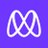Microverse

Microverse is an online school for remote software developers. The company offers a full-time technical curriculum that helps students around the world develop their technical skills, build projects, and improve the soft skills they need to excel in remote teams. It was founded in 2018 and is based in San Francisco, California.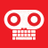Resilient Coders

Resilient Coders is a volunteer-based program focused on making web technology more available to urban youth who might not otherwise be exposed to it. It's a three-part program that funnels students from learning HTML after school, through our downtown "Coworking" sessions, and ultimately, hourly employment.Scaler

Scaler provides an e-learning platform and interview preparation website for software professionals. The company offers courses on machine learning, data structure, javascript, and more. It was formerly known as InterviewBit Academy. The company was founded in 2019 and is based in Bangalore, India.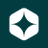Andela

Andela provides an integrated platform to access a global talent network. It allows users to enhance skills and pairs with companies as full-time, distributed team members. It was founded in 2014 and is based in New York, New York.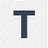Toptal

Toptal connects start-ups, businesses, and organizations to a growing network of developers in the world. Toptal provide companies with software engineers and teams on an hourly, part-time, or full-time basis for any web, mobile, or desktop technology stack.

## Discover the right solution for your team

The CB Insights tech market intelligence platform analyzes millions of data points on vendors, products, partnerships, and patents to help your team find their next technology solution.CBI websites generally use certain cookies to enable better interactions with our sites and services. Use of these cookies, which may be stored on your device, permits us to improve and customize your experience. You can read more about your cookie choices at our privacy policy here. By continuing to use this site you are consenting to these choices.